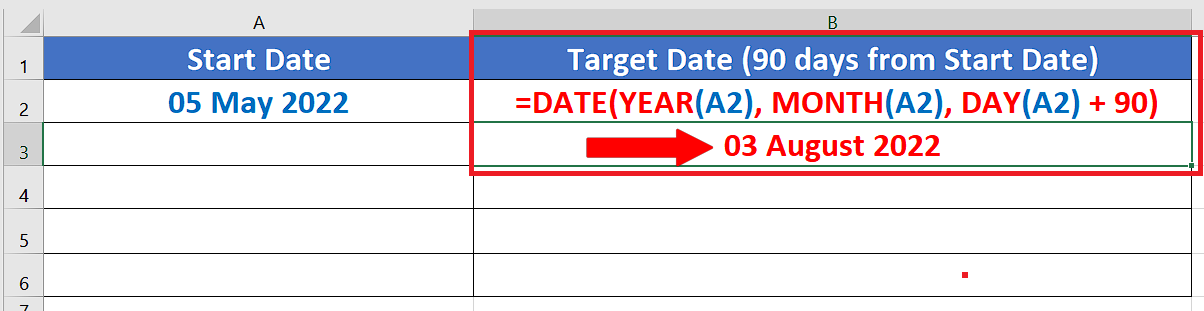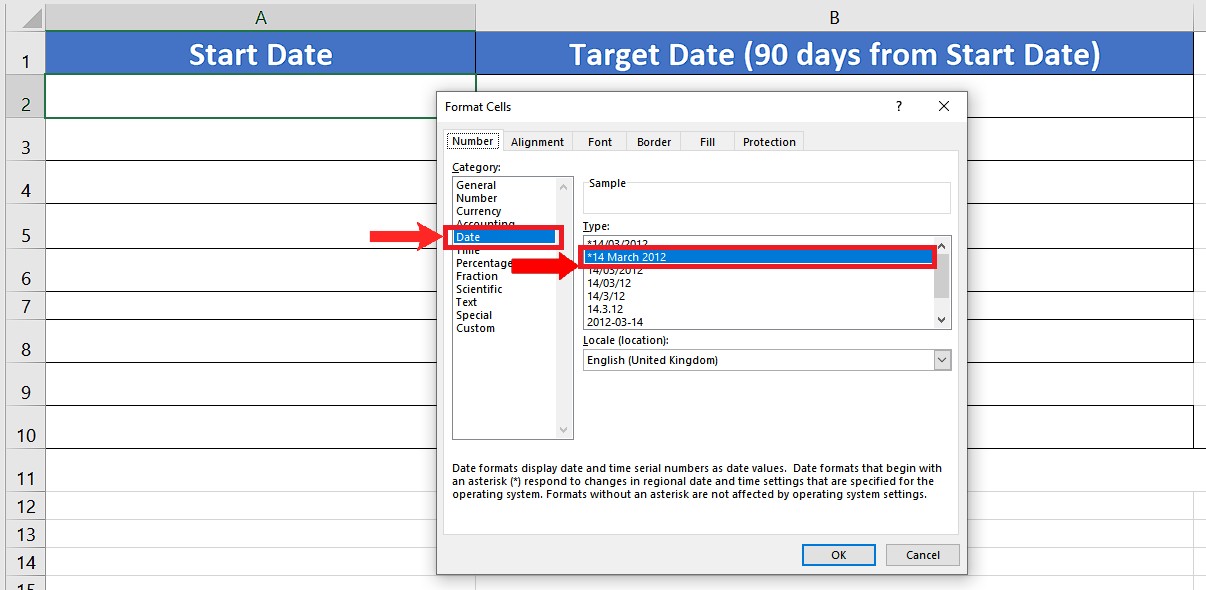# How to get to the date of 90 days from 5 may 2022 in ExcelWhile we are working with dates in excel, often we have to add or subtract the dates from each other. Sometimes we want to calculate a specific date (past or future) with reference to another date in our data set. So let’s look at a specific example in which we’ll find a date which is 90 days (3 months) after a specific date i.e. 5 May 2022. Let’s see how to do this step by step;

### Step 1 – Choose a friendly date format– First we’ll choose a friendly date format to understand the date easily. To do this select a cell in which you wish to display the current date and press CTRL+1.
– This will open up the format cell menu and use this formula as shown above.

### Step 2 – Create and implement the formula to find the date 90 days from desired date– To find the date which is 90 days from the desired date i.e. 5 May 2022 we’ll have to use Excel’s built-in DATE formula. To do this select a cell in which you wish to find the future date (after 90 days) and implement this formula shown below;
=DATE(YEAR(A2),MONTH(A2),DAY(A2)+ 90)

Let’s implement this formula as shown in above picture.

## Break down of the formula:

We used the DATE function of Excel and the format of the DATE function is as follows;

DATE (year, month, day)

This function is used to create a date using a given year, month and day. When we passed on A2 (holding today’s date) as a parameter to this function in each argument then it used the year ofA2 (2022), month of A2 (May) and day of A2 (5) as well to create a date. However, we wanted to find out a date which is 90 days from 5 May 2022, so we added 90 days in the day section of the formula. This is how we found out a date that is 90 days in the future from today. Using this technique we can find any date in the past or future with reference to any specific date.